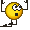# Differentiability of a function between manifolds

• A
• Maxi1995

#### Maxi1995

Hello,
let $$M^n \subset \mathbb{R}^N$$ $$N^k \subset \mathbb{R}^K$$
be two submanifolds.
We say a function $$f : M \rightarrow N$$ is differentiable if and only if for every map $$(U,\varphi)$$ of M the transformation

$$f \circ \varphi^{-1}: \varphi(U) \subset \mathbb{R}^N \rightarrow \mathbb{R}^K$$

is differentiable.

Does this mean that it is differentiable in the sense of a "normal" function in several variables, thus to say $$d(f \circ \varphi^{-1})(x)=df(\varphi^{-1}(x))d\varphi^{-1}(x)$$?

You do not have ##df(\ldots)## while are defining it. You transform ##f## to ##g=f\circ \varphi^{-1}## which is a real valued function which you know how to differentiate. Thus you have ##dg=df\,d\varphi^{-1}## which defines you ##df##.

Thank you very much.